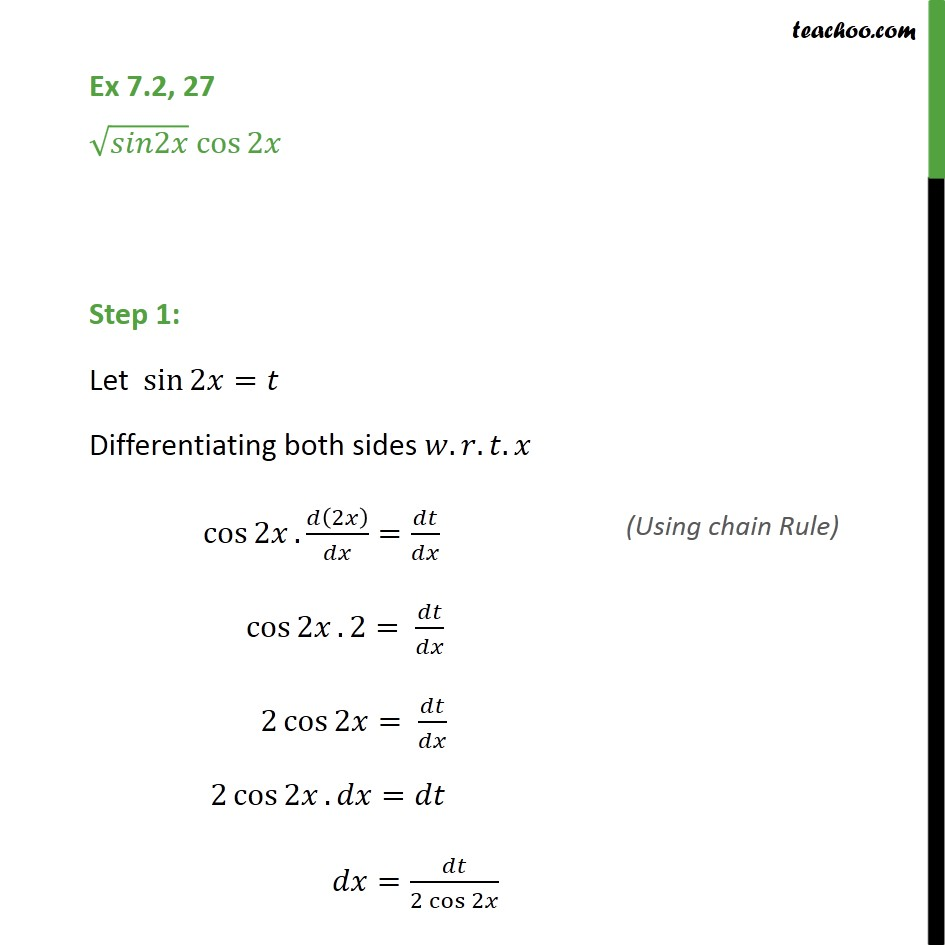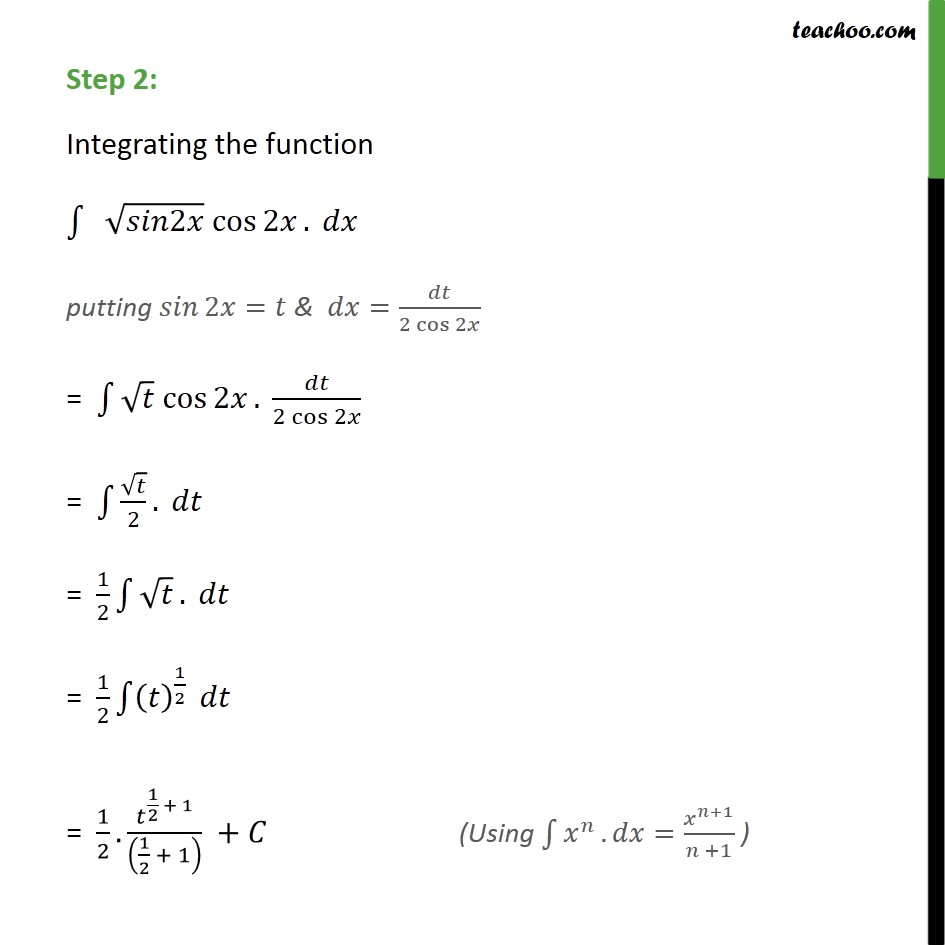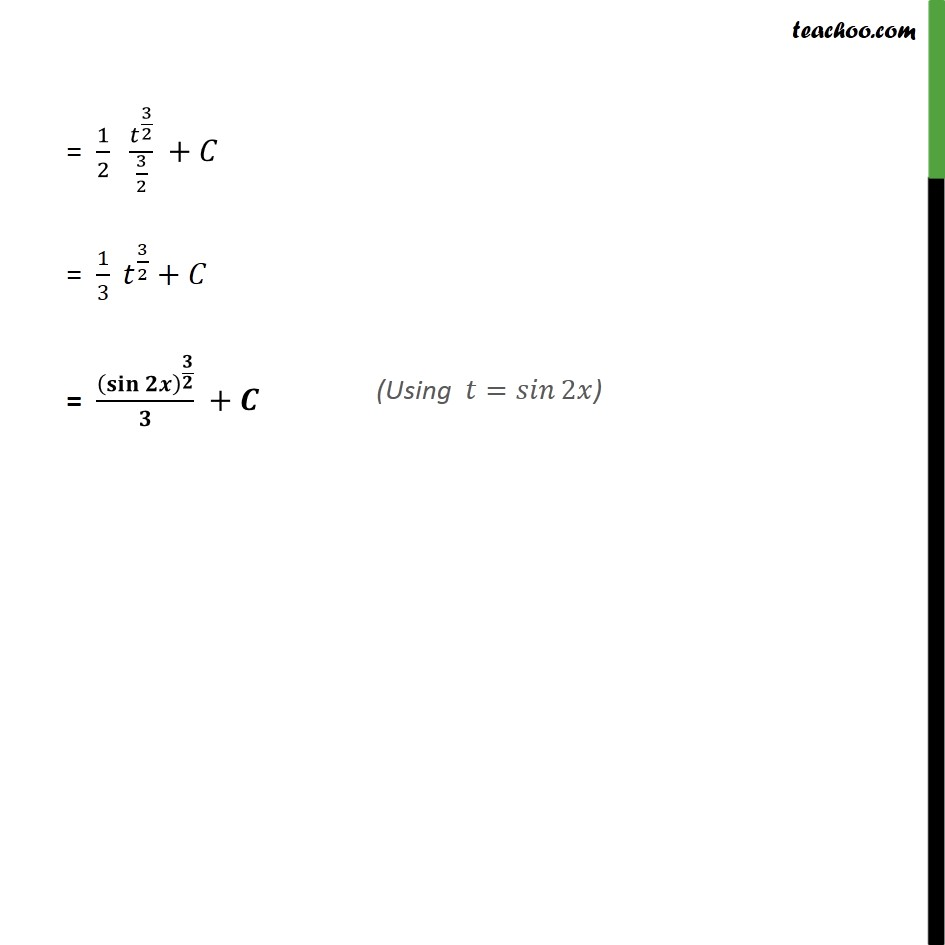Integration Full Chapter Explained - Integration Class 12 - Everything you need1. Chapter 7 Class 12 Integrals
2. Serial order wise
3. Ex 7.2

Transcript

Ex 7.2, 27 2 cos 2 Step 1: Let sin 2 = Differentiating both sides . . . cos 2 . 2 = cos 2 . 2= 2 cos 2 = 2 cos 2 . = = 2 cos 2 Step 2: Integrating the function 2 cos 2 . putting 2 = & = 2 cos 2 = cos 2 . 2 cos 2 = 2 . = 1 2 . = 1 2 1 2 = 1 2 . 1 2 + 1 1 2 + 1 + = 1 2 3 2 3 2 + = 1 3 3 2 + = +

Ex 7.2

Chapter 7 Class 12 Integrals
Serial order wise

About the AuthorDavneet Singh
Davneet Singh is a graduate from Indian Institute of Technology, Kanpur. He has been teaching from the past 9 years. He provides courses for Maths and Science at Teachoo.